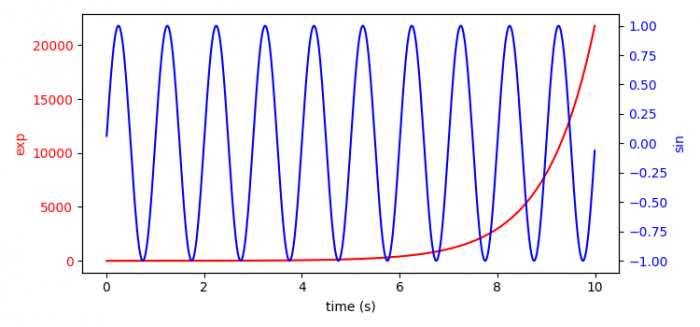# How to plot with different scales in Matplotlib?

To plot with different scales in matplotlib, we can take the following steps −

## Steps

• Set the figure size and adjust the padding between and around the subplots.
• Create t, data1 and data2 data points using numpy
• Create a figure and a set of subplots, ax1.
• Initialize a color variable.
• Set x and y labels of axis 1.
• Plot t and data1 using plot() method.
• Set label colors using tick_params() method.
• Create a twin Axes sharing the X-axis, ax2.
• Perform steps 4, 6, 7 with a different dataset on axis 2.
• To display the figure, use show() method.

## Example

import numpy as np
import matplotlib.pyplot as plt

plt.rcParams["figure.figsize"] = [7.50, 3.50]
plt.rcParams["figure.autolayout"] = True

t = np.arange(0.01, 10.0, 0.01)
data1 = np.exp(t)
data2 = np.sin(2 * np.pi * t)
fig, ax1 = plt.subplots()
color = 'red'

ax1.set_xlabel('time (s)')
ax1.set_ylabel('exp', color=color)
ax1.plot(t, data1, color=color)
ax1.tick_params(axis='y', labelcolor=color)
ax2 = ax1.twinx()

color = 'blue'
ax2.set_ylabel('sin', color=color)
ax2.plot(t, data2, color=color)
ax2.tick_params(axis='y', labelcolor=color)

plt.show()

## Output